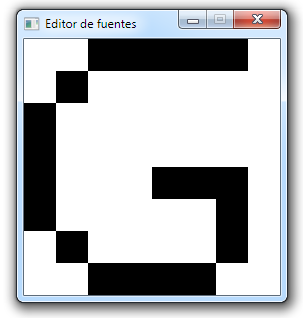# Gzalo

ES · EN

## Introduction to SDL2, developing a pixel font editor

This small tutorial will show how to develop a small 8x8 pixel font editor, useful for designing small fonts with a pixel style. C++ y SDL2 will be used. C/C++ knowledge is recommended.We first need to include all the header files (.h extension) of all the libraries that we’ll use in the editor. These inclusions are required so that the compiler can correctly reference the functions we’ll use.

``````#include <SDL2/SDL.h>
#include <fstream>
#include <iostream>
using namespace std;
``````
``````int main(int argc, char* args[]){
if(SDL_Init(SDL_INIT_EVERYTHING) != 0){
cerr << "Error init SDL" << endl;
return -1;
}
``````

We declare the main function of the program, and try to initialize the library. If it fails, we print an error and return

``````	int pixels = {};
``````

We declare a 2D array of integers, which will contain the values of each cell (1 if cell is black, 0 if cell is white). To keep things simple, every glyph size will be fixed at 8x8. By default all of them will be intialized with the value 0.

``````	SDL_Surface *window = SDL_CreateWindow("Font editor", SDL_WINDOWPOS_UNDEFINED, SDL_WINDOWPOS_UNDEFINED, 8 * 32,
8 * 32, SDL_WINDOW_SHOWN);

if(screen == NULL){
cerr << "Error creating window" << endl;
return -1;
}

``````

We try to create a 256x256 pixel window with the given title. If that fails, we print an error.

``````
bool closed=false;
int buttonPressed = 0;

``````

We declare two variables, one bool that will indicate if the program is still running and the other one that indicates if any mouse button is down (0=none, 1=left, 2=right).

``````	while(!quit){
``````

While the window is still open, we keep looping.

``````	SDL_Event event;
while(SDL_PollEvent(&event)){
``````

While there are available events, we process them.

``````if(event.type == SDL_QUIT)
quit = true;
``````

If the X of the window got pressed we proceed to quit in the next frame, by setting the variable `quit` to true.

``````if(event.type == SDL_MOUSEBUTTONDOWN){
if(event.button.button == SDL_BUTTON_LEFT)
pressedButton = 1;
else if(event.button.button == SDL_BUTTON_RIGHT)
pressedButton = 2;
}
``````

If a button got pressed, we remember which button it was.

``````if(event.type == SDL_MOUSEBUTTONUP)
pressedButton = 0;
``````

If a button got released, we remember it.

``````if(pressedButton != 0){
int posX = event.motion.x;
int posY = event.motion.y;
``````

If there is a button press, we store the position of the mouse.

``````		if(posX<32*8 && posY<32*8){
int cellX = posX/32;
int cellY = posY/32;

if(pressedButton == 1){
pixels[cellY][cellX] = 1;
}else{
pixels[cellY][cellX] = 0;
}
}

}
}
``````

If the mouse is inside the window, we calculate to which cell that position corresponds, by dividing the position by the cell size (32 px in this case). If the left button is pressed, we set a value of 1, otherwise a 0.

``````		SDL_Surface *screenSurface = SDL_GetWindowSurface(window);

for(int y=0;y<8;y++){
for(int x=0;x<8;x++){

SDL_Rect rect;
rect.x = x*32;
rect.y = y*32;
rect.h = 32;
rect.w = 32;

``````

We get a surface that can be used to draw on the created window. We then iterate through the 64 cells and calculate the position of each square.

``````				if(font[y][x]==0){
SDL_FillRect(screen,&rect,0x00FFFFFF);
}else{
SDL_FillRect(screen,&rect,0x00000000);
}
}
}
``````

If the value of the cell was in 0, we paint the corresponding rectangle with white, otherwise we paint it black.

``````		SDL_UpdateWindowSurface(screen);
SDL_Delay(1);
}
``````

We force the window to redraw and wait some time to avoid hogging the CPU.

``````	ofstream of("output.txt");
for(int y=0;y<8;y++){
for(int x=0;x<8;x++){
of << font[y][x] << ",";
}
}
of.close();
``````

If we got outside the loop, it’s because the app got closed, so we open the file “output.txt” as output, and we save the state of each cell. Finally, we close the file.

``````	SDL_Delay(100);
``````

We wait a while and we open the file with notepad. It’s not a very elegant solution but it works correctly, and allows for a fast copy of the values of the pixels.

``````	SDL_DestroyWindow(window);
SDL_Quit();
return 0;
}
``````

We destroy the created window, and then tell SDL to free every piece of memory it has used, and we tell the operating system that the process finished correctly.

The source code contains a `CMakeLists.txt` file, that can be used by an IDE such as IntelliJ CLion to compile the code using any C++ compiler installed in your system. Before actually configuring `cmake`, your enviroment must have the SDL2 library installed (the `-devel` version, which includes the headers and lib files).